Described

Calculate perimeter of the circle described by a triangle with sides 478, 255, 352.

Result

p =  1539.5

Solution:Leave us a comment of example and its solution (i.e. if it is still somewhat unclear...):Be the first to comment!To solve this example are needed these knowledge from mathematics:

Do you want to convert length units? See also our trigonometric triangle calculator.

Next similar examples:

1. Circle inscribed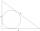Calculate the perimeter and area of a circle inscribed in a triangle measuring 3 , 4 and 5 cm.
2. TriangleCalculate heights of the triangle ABC if sides of the triangle are a=75, b=84 and c=33.
3. Triangle SAAThe triangle has one side long 71 m and its two internal angles is 60°. Calculate the perimeter and area of the triangle.
4. Circles 2Calculate the area bounded by the circumscribed and inscribed circle in triangle with sides 12 cm, 14 cm, 18 cm.
5. AceThe length of segment AB is 24 cm and the point M and N divided it into thirds. Calculate the circumference and area of this shape.
6. Circular lawnAround a circular lawn area is 2 m wide sidewalk. The outer edge of the sidewalk is curb whose width is 2 m. Curbstone and the inner side of the sidewalk together form a concentric circles. Calculate the area of the circular lawn and the result round to 1
7. Sss triangleCalculate the area and heights in the triangle ABC by sides a = 8cm, b = 11cm, c = 12cm
8. DiamondRhombus has side 17 cm and and one of diagonal 22 cm long. Calculate its area.
9. Circles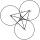Three circles of radius 95 cm 78 cm and 64 cm is mutually tangent. What is the perimeter of the triangle whose vertices are centers of the circles?
10. Silo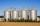Outer perimeter of silo is 32 m. Concrete wall is 35 cm thick. What is diameter and area of inside floor of silo?
11. Estate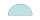Semicircle estate must be fence. The straight section has 26 meters long fence. How many meters of fence should buy?
12. Equilateral triangle v2Equilateral triangle has a perimeter 36 dm. What is its area?
13. Park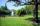In the newly built park will be permanently placed a rotating sprayer irrigation of lawns. Determine the largest radius of the circle which can irrigate by sprayer P so not to spray park visitors on line AB. Distance AB = 55 m, AP = 36 m and BP = 28 m.
14. TriangleDetermine whether a triangle can be formed with the given side lengths. If so, use Heron's formula to find the area of the triangle. a = 158 b = 185 c = 201
15. The farmerThe farmer would like to first seed his small field. The required amount depends on the seed area. Field has a triangular shape. The farmer had fenced field, so he knows the lengths of the sides: 119, 111 and 90 meters. Find a suitable way to determine th
16. Center traverseIt is true that the middle traverse bisects the triangle?
17. Theorem proveWe want to prove the sentence: If the natural number n is divisible by six, then n is divisible by three. From what assumption we started?